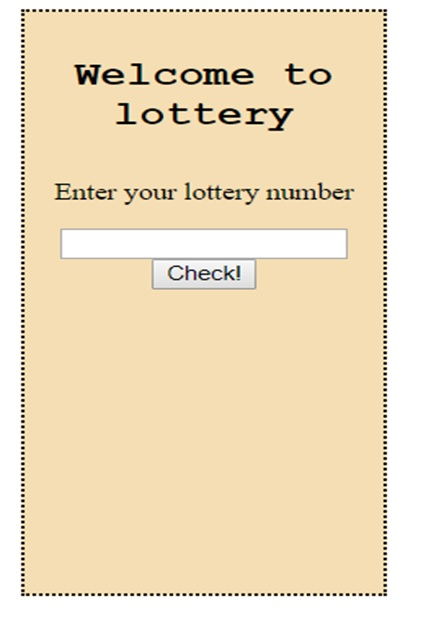# JavaScript Math Object (Methods and Examples)

JavaScript Math Object: In this tutorial, we are going to learn about the Math object and its methods with examples in JavaScript.
Submitted by Siddhant Verma, on October 03, 2019

## JavaScript Math Object

Math is an object inbuilt in the JavaScript library that has various methods we as a programmer can use for carrying out mathematical operations.

Open the chrome dev console to try out the examples by right-clicking on the browser → selecting inspect → selecting console or simply type f12.

You can see various properties attached to this object by simply logging out on the console Math.

```    console.log(Math);
```

It also contains very precise values of some mathematical constants such as pi, Euler's number, square root of 2, etc.

It's pretty simple to use it. Let's start by seeing some constants on the console.

```console.log(Math.PI);
console.log(Math.LOG2E);
console.log(Math.LN2);
```

### Math.round() method

We can use the round() method of this object to round any number to the nearest integer.

```console.log(Math.round(5.2)); //5
console.log(Math.round(5.7)); //6
console.log(Math.round(0.1)); //0
```

### Math.floor() method

Much similar to round is floor(), which gives an integer smaller than the number passed to it.

```console.log(Math.floor(2.33)); //2
console.log(Math.floor(2.99)); //2
console.log(Math.floor(11.74)); //11
```

### Math.ceil() Method

ceil() is something that does totally opposite of floor. It gives an integer greater than the number passed to it.

```console.log(Math.ceil(43.44)); //44
console.log(Math.ceil(9.1)); //10
console.log(Math.ceil(12.8)); //13
```

### Math.trunc() Method

If we simply want the integer part of the number and neglect the decimal, we can use trunc().

```console.log(Math.trunc(5.667)); //5
console.log(Math.trunc(0.9359)); //0
```

### Math.random() Method

Math.random() gives us a random number between 0 and 1. Every time the method is invoked, a new random number is generated.

```console.log(Math.random());
```

Okay so now let's build a simple program to see where we can utilize Math objects in real-world scenarios. Let's say we have to generate a random number for a lottery. We have to generate a random winning ticket in a particular range. We can set the range to initially a smaller value to test our lottery app.

All you need is an index.html so go ahead and create an index.html file in any folder and copy the following started template,

All we have done till now is created something that looks like this:

```<html lang="en">

<meta charset="UTF-8">
<meta name="viewport" content="width=device-width, initial-scale=1.0">
<meta http-equiv="X-UA-Compatible" content="ie=edge">

<title>Lottery Game</title>

<style>
body {
text-align: center;
}

div {
border: 2px dotted black;
background: wheat;
height: 400px;
width: 200px;
display: inline-block;
}

h2 {
text-align: center;
font-family: 'Courier New', Courier, monospace
}
</style>

<body>
<div class="main">
<h2>Welcome to lottery</h2>
<input type="text" name="Maximum lottery" id="lottery">
<button class="btn">Check!</button>
</div>
</body>
<script>
</script>

</html>
```

OutputNow we simply need to capture the input value and check it against a randomly generated number. If they're both the same, the player wins the lottery otherwise he does not.

```<script>
var lotteryNo = document.querySelector('#lottery');
var btn = document.querySelector('.btn');
var range = 10;
var winningTicket = Math.floor(Math.random() * 10);

console.log(lotteryNo.value, winningTicket);
if (winningTicket == lotteryNo.value)
else
alert('Sorry you lost. Better luck next time!')
})

</script>
```

That's it! Right now we're only generating lottery numbers from 1 to 10. You can increase the range to 100 or 1000 or whatever you like. This is a very basic and simple implementation of the random() method in Math object.

There are other methods such as pow() which calculates a to the power b, cos(), sin(), min(), max() which are quite self-explanatory.

```console.log(Math.sqrt(64)); //8
console.log(Math.min(4,7,99)); //4
console.log(Math.max(9,-11,23)); //23
```

A great way to implement most of the methods of the Math Object is to make your very own calculator app.

Languages: » C » C++ » C++ STL » Java » Data Structure » C#.Net » Android » Kotlin » SQL
Web Technologies: » PHP » Python » JavaScript » CSS » Ajax » Node.js » Web programming/HTML
Solved programs: » C » C++ » DS » Java » C#
Aptitude que. & ans.: » C » C++ » Java » DBMS
Interview que. & ans.: » C » Embedded C » Java » SEO » HR
CS Subjects: » CS Basics » O.S. » Networks » DBMS » Embedded Systems » Cloud Computing
» Machine learning » CS Organizations » Linux » DOS
More: » Articles » Puzzles » News/Updates